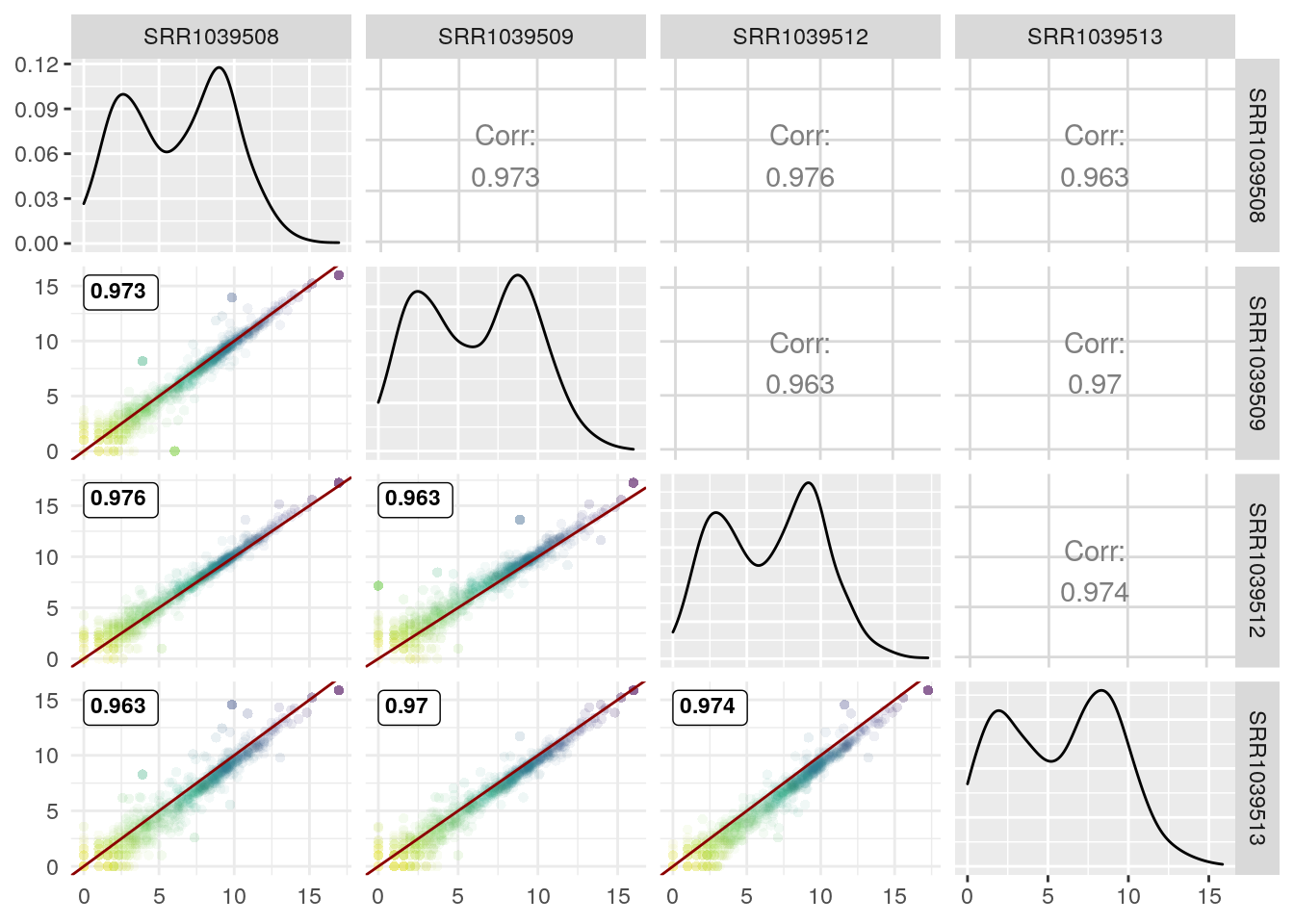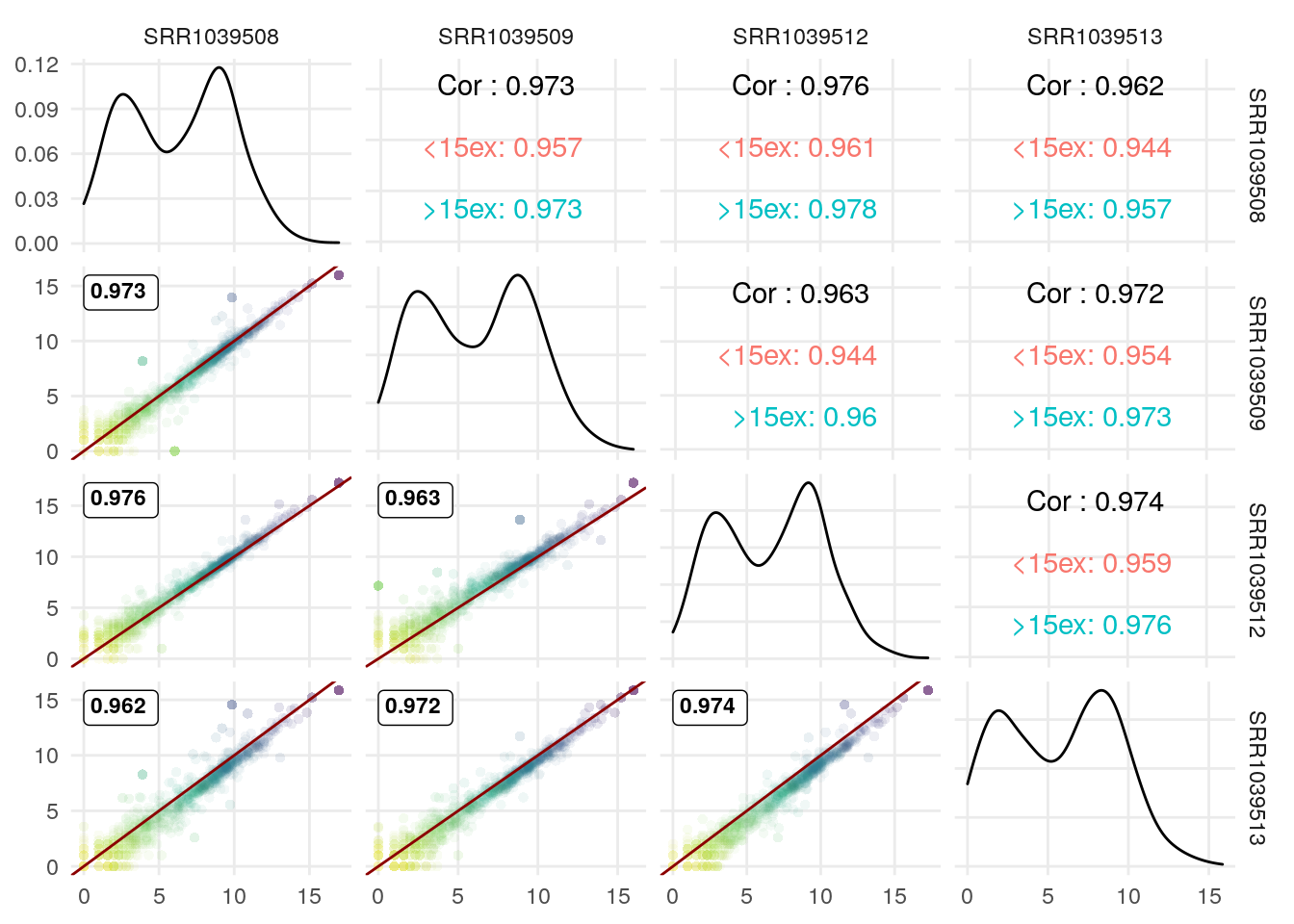# Nicer scatter plots in GGgally ggpairs-ggduo

GGally is an extension of ggplot2. I use it mainly as a replacement/refinement of pairs in base R.

Here, I want to replace the default scatter plot used to compare 2 continuous variables with something nicer, especially adapted to plotting large number of points.

Load the library and some RNA-seq data:

``````library(GGally)
library(airway)
data(airway)
``````

The airway dataset contains data for 4 cell lines treated or not with dexamethasone:

``````colData(airway)[,1:3]
``````
SampleName cell dex
SRR1039508 GSM1275862 N61311 untrt
SRR1039509 GSM1275863 N61311 trt
SRR1039512 GSM1275866 N052611 untrt
SRR1039513 GSM1275867 N052611 trt
SRR1039516 GSM1275870 N080611 untrt
SRR1039517 GSM1275871 N080611 trt
SRR1039520 GSM1275874 N061011 untrt
SRR1039521 GSM1275875 N061011 trt

Let’s keep a fraction of the data for simplicity

``````df <- as.data.frame(assays(airway)\$counts[,1:4]) #first 4 columns
df <- df[rowSums(df)>4,] #keep genes with some counts
set.seed(123)
df <- df[sample.int(nrow(df),1e3),] #random 1K genes
``````

default behavior of `GGally::ggpair`:

``````GGally::ggpairs(log2(df+1))
``````Here is a better function to make the scatterplots in the lower triangle. It is largely based on this blog post and implements:

• viridis coloring of points based on expression level (practically based on effect size captured by the first principal component). I added some code to get consistent coloring even if orientation of the first PC differs between pairs of columns.
• `alpha` based on inverse of 2D density, i.e. low alpha when there are lots of points and higher when points are more scattered

Also, the use of `GGally:eval_data_col` is described here:

``````GGscatterPlot <- function(data, mapping, ...,
method = "spearman") {

#Get correlation coefficient
x <- GGally::eval_data_col(data, mapping\$x)
y <- GGally::eval_data_col(data, mapping\$y)

cor <- cor(x, y, method = method)
#Assemble data frame
df <- data.frame(x = x, y = y)
# PCA
nonNull <- x!=0 & y!=0
dfpc <- prcomp(~x+y, df[nonNull,])
df\$cols <- predict(dfpc, df)[,1]
# Define the direction of color range based on PC1 orientation:
dfsum <- x+y
colDirection <- ifelse(dfsum[which.max(df\$cols)] <
dfsum[which.min(df\$cols)],
1,
-1)
#Get 2D density for alpha
dens2D <- MASS::kde2d(df\$x, df\$y)
df\$density <- fields::interp.surface(dens2D ,
df[,c("x", "y")])

if (any(df\$density==0)) {
mini2D = min(df\$density[df\$density!=0]) #smallest non zero value
df\$density[df\$density==0] <- mini2D
}
#Prepare plot
pp <- ggplot(df, aes(x=x, y=y, color = cols, alpha = 1/density)) +
ggplot2::geom_point(shape=16, show.legend = FALSE) +
ggplot2::scale_color_viridis_c(direction = colDirection) +
#                scale_color_gradient(low = "#0091ff", high = "#f0650e") +
ggplot2::scale_alpha(range = c(.05, .6)) +
ggplot2::geom_abline(intercept = 0, slope = 1, col="darkred") +
ggplot2::geom_label(
data = data.frame(
xlabel = min(x, na.rm = TRUE),
ylabel = max(y, na.rm = TRUE),
lab = round(cor, digits = 3)),
mapping = ggplot2::aes(x = xlabel,
y = ylabel,
label = lab),
hjust = 0, vjust = 1,
size = 3, fontface = "bold",
inherit.aes = FALSE # do not inherit anything from the ...
) +
theme_minimal()

return(pp)
}
``````

Now use in ggpairs (adjust correlation method to be consistent with defaults in GGscatterPlot):

``````GGally::ggpairs(log2(df+1),
1:4,
lower = list(continuous = GGscatterPlot),
upper = list(continuous = wrap("cor", method= "spearman")))
``````Now with Pearson correlation coefficient (and removing the correlation coefficient in the upper triangle since we already have them in the lower triangle):

``````GGally::ggpairs(log2(df+1),
1:4,
lower = list(continuous = wrap(GGscatterPlot, method="pearson")),
upper = "blank") +
theme_minimal() +
theme(panel.grid.minor = element_blank())
``````We can use the upper triangle to plot other info since the scatter plots include the correlation coefficient.
I illustrate it here with the correlation coefficient for genes with >15 exons vs those with <15 exons:

``````exonNumber <- elementNROWS(rowRanges(airway[rownames(df),]))
df\$MoreThan15Exons <- ifelse(exonNumber>15,
">15ex", "<15ex")
df[,1:4] <- log2(df[,1:4]+1)

GGally::ggpairs(df,
1:4,
lower = list(continuous = wrap(GGscatterPlot, method="pearson")),
upper = list(continuous = wrap(ggally_cor, alignPercent = 0.8),
mapping = ggplot2::aes(color = MoreThan15Exons))) +
theme_minimal() +
theme(panel.grid.minor = element_blank())
``````The `GGscatterPlot` function can also be used with `GGally::ggduo` to study the link between 2 sets of columns/samples. Here an example comparing control vs DEX samples:

``````GGally::ggduo(df,
c(1,3),
c(2,4),
types = list(continuous = GGscatterPlot)) +
theme_minimal() +
theme(panel.grid.minor = element_blank())
``````##### Pascal GP Martin
###### Senior Research Specialist (IR1) at INRAE

Research Specialist in Genomics and Bioinformatics